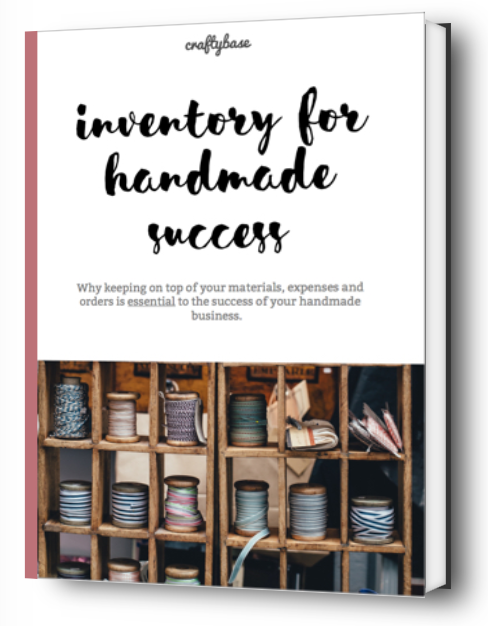bookkeeping tax

# What is the Weighted Average Cost Method?

Although most people learned how to calculate a simple average from their high school days, weighted averages are not something that many people have seen or needed to calculate directly. You have however most likely been impacted by weighted averages without perhaps knowing it.In perpetual inventory systems like Craftybase, weighted averages are used to provide a high level of accuracy to your material and product costings.

Although most people learned how to calculate a simple average from their high school days, weighted averages are not something that many people have seen or needed to calculate directly. You have however most likely been impacted by weighted averages without perhaps knowing it.

One common example of weighted averages is school grades: when calculating your final grade for the year, most teachers will apply different weightings to each of your assignments and tests - they don’t just simply add up each score and divide it by the number of tests you were given. Instead a weighting of relative effort is usually applied to each testable item - this ensures that final exams or major assignments are factored into your final grade in a much bigger way than weekly quizzes and other minor homework tasks.

The definition of a weighted average is therefore an average that has been assigned a relative importance (“weight”). Why is a weighted average important for inventory? Essentially, the average will only find the “middle” value regardless of any other factors - this can be problematic for inventory calculations as you will have situations where your stock value will change throughout the year based on how much you buy and what unit price you purchase it for. Weighted averages factors in these cost differences each time you purchase the material.

Going back to our school grades example, the weighting used was “relative effort”. In inventory accounting, weighting is given based on the quantity of stock available at a certain point in time.

Let’s work through a simple example. Let’s say you have two units of a material: one unit was purchased at \$10 and another unit was purchased later on at \$20.

As a quick reminder, the formula to use to calculate an average from a set of numbers is:

The average cost of the material in our example above is therefore:

(\$10 + \$20) ÷ 2 = \$15

Let’s now calculate an example where there are different quantities of each unit cost involved. If one unit of your material was purchased at \$10, but two units were purchased at \$20 then we should expect the average should go slightly up:

(\$10 + \$20 + \$20) ÷ 3 = \$16.60

The average is now slightly higher. This is due to the extra weighting placed on the two \$20 items as there were more of them to consider.

For perpetual inventory weighted averages, this calculation is constantly (or “perpetually”) calculated whenever a stock change is made to your material’s inventory. This is known as a rolling or moving average (as it changes as you move forward in time).

This ensures that the calculation factors in the current quantity available and the cost of each every time. This is quite understandably a tedious calculation to make, which is why you will most likely be using an inventory system that does this automatically for (like Craftybase) rather than doing this yourself by hand.

Let’s now look at the example above represented as stock changes (purchases only) in an inventory system:

Date Purchased Unit Cost Quantity New Stock on Hand New Average Unit Cost
1 Jan \$10 1 \$10 \$10
3 Jan \$20 2 \$10 + \$20 + \$20 \$16.60

If I was to take my stock value on the 1st Jan, I would have a value of \$10. On the 3rd Jan, I add a new purchase to my inventory system which then triggers a recalculation of the average to \$16.60.

To add to this, remember that each and every stock change in the perpetual inventory system will recalculate your average. Let’s record the dates of usage of materials as well as purchases and see what happens to the averaging:

Date Unit Cost Quantity Change New Stock on Hand New Average Unit Cost
1 Jan \$10 +1 \$10 \$10.00
3 Jan \$20 +2 \$10 + \$20 + \$20 \$16.60
4 Jan \$20 -1 \$10 + \$20 \$15.00

In an inventory system, rolling averages are used not only for material unit costings but also for the overall cost of manufacturing (or purchasing) the goods you sell: this allows the system to be able to calculate your inventory value and COGS.

In the product example, making the item increases your stock and selling the item decreases your stock: the cost to make the stock is therefore calculated when either of these things occur.

More details on how COGS (Cost of Goods Sold) can be found here How do I calculate Cost of Goods Sold (COGS)?

For more information on how Weighted Average Cost differs to other Inventory Cost methods such as LIFO and FIFO, please see our blog post here: Which method of valuing inventory is best for your handmade business?

It's a fact: inventory tracking improves both your business and your bottom line. Our eBook introduces online craft sellers to the absolute basics of inventory in an easy to understand way, giving you the knowledge you need to finally get your inventory, costs and taxes under control.